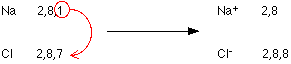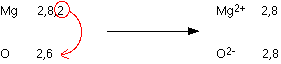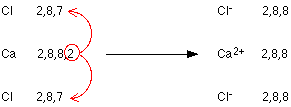## Welcome!Welcome to Nolver's Science Corner! If you would like to read my blog, visit my website on Nolver's Cozy Corner. This page is dedicated to people who are into scientific subjects. Don't hesitate to be a member of this page!

For tags, visit my website on Nolver's Pretty Tags, for PSP tubes, visit my page on Nolver's Cool PSP Tubes. For my personal blog, visit my page on Nolver's Room.

**Always scroll down to read the actual post**©2013-2020 Nolver's Science Corner. Images with my watermark are copyrighted by me, so I reserve all rights. It is not allowed to edit or to modify the pictures in any way. It is not allowed to use the images/backgrounds from the layout and from the welcome box. Please respect my work.

## Thursday, February 28, 2013

### Ionic (electrovalent) bondingA simple view of ionic bonding

The importance of noble gas structures

At a simple level (like GCSE) a lot of importance is attached to the electronic structures of noble gases like neon or argon which have eight electrons in their outer energy levels (or two in the case of helium). These noble gas structures are thought of as being in some way a "desirable" thing for an atom to have.

You may well have been left with the strong impression that when other atoms react, they try to organise things such that their outer levels are either completely full or completely empty.

Ionic bonding in sodium chloride

Sodium (2,8,1) has 1 electron more than a stable noble gas structure (2,8). If it gave away that electron it would become more stable.

Chlorine (2,8,7) has 1 electron short of a stable noble gas structure (2,8,8). If it could gain an electron from somewhere it too would become more stable.

The answer is obvious. If a sodium atom gives an electron to a chlorine atom, both become more stable.The sodium has lost an electron, so it no longer has equal numbers of electrons and protons. Because it has one more proton than electron, it has a charge of 1+. If electrons are lost from an atom, positive ions are formed.

Positive ions are sometimes called cations.

The chlorine has gained an electron, so it now has one more electron than proton. It therefore has a charge of 1-. If electrons are gained by an atom, negative ions are formed.

A negative ion is sometimes called an anion.

The nature of the bond

The sodium ions and chloride ions are held together by the strong electrostatic attractions between the positive and negative charges.

The formula of sodium chloride

You need one sodium atom to provide the extra electron for one chlorine atom, so they combine together 1:1. The formula is therefore NaCl.

Some other examples of ionic bonding

magnesium oxideAgain, noble gas structures are formed, and the magnesium oxide is held together by very strong attractions between the ions. The ionic bonding is stronger than in sodium chloride because this time you have 2+ ions attracting 2- ions. The greater the charge, the greater the attraction.

The formula of magnesium oxide is MgO.

calcium chlorideThis time you need two chlorines to use up the two outer electrons in the calcium. The formula of calcium chloride is therefore CaCl2.

potassium oxideAgain, noble gas structures are formed. It takes two potassiums to supply the electrons the oxygen needs. The formula of potassium oxide is K2O.

Source: http://www.chemguide.co.uk

## Monday, February 18, 2013

### ThunderstormThe emergence of storm has to do with the soaring and descending air currents in large rain clouds and the electric tension of earth and atmosphere. Concentrations of electric charges may arise by the strong air currents. In storm clouds the strong soaring warm air and descending cold air flow closely past each other with maximum speeds of 100 kilometer per hour. With these currents, electrically charged particles are being carried whereby the cloud is being charged like a huge capacitor. Thereby discharges are possible between the cloud and other clouds or between the cloud and the earth, which leads to lightning and thunder.

Source: www.knmi.nl

## Sunday, February 10, 2013

### Dried Plums: A Natural Way to Treat ConstipationTreating Constipation Naturally with Dried Plums

Some people use psyllium to treat constipation. Psyllium consists of husks from the Plantango ovate plant and is the active ingredient in such products as Metamucil and Colon Cleanse. Psyllium is effective for treating constipation, because it's a rich source of fiber - but there's an even better solution.

According to a study published in Alimentary Pharmacology and Therapeutics, dried plums are better than psyllium for perking up sluggish bowels. When they gave 40 volunteers either 11 grams of psyllium or 50 grams of dried plums (prunes) daily for 3 weeks, those who munched on prunes had more bowel movements. In fact, the group who ate the dried plums had 3.5 additional bowel movements each week compared to only 2.8 in the psyllium group. They also had a healthier stool consistency.

Treating Constipation with Dried Plums: Why Does It Work?

Prunes are not only a good source of fiber, they also contain sorbitol, a natural sugar alcohol that draws water into the intestines and helps soften the stool - which is a good thing if you're constipated. Researchers believe it's the sorbitol and components in dried plums other than fiber that make it superior to psyllium for treating constipation.

Other Health Benefits of Plums

There are good reasons to eat dried plums - other than to treat constipation. They have as much antioxidant power as the blueberry, and they're a good source of vitamin A, vitamin C and potassium. Plus, most people enjoy eating dried plums more than drinking a glassful of psyllium in water or juice.

Treating Constipation with Dried Plums: The Bottom Line?

Next time you're constipated, skip the drugstore and head to your local supermarket instead. Pick up some dried plums and eat them once a day. If it doesn't relieve your constipation in a few days, see your doctor. Constipation can sometimes be a symptom of more serious medical problems.

Source: http://voices.yahoo.com

### A little bit of religion and maths for you1 x 8 + 1 = 9

12 x 8 + 2 = 98

123 x 8 + 3 = 987

1234 x 8 + 4 = 9876

12345 x 8 + 5 = 98765

123456 x 8 + 6 = 987654

1234567 x 8 + 7 = 9876543

12345678 x 8 + 8 = 98765432

123456789 x 8 + 9 = 987654321

1 x 9 + 2 = 11

12 x 9 + 3 = 111

123 x 9 + 4 = 1111

1234 x 9 + 5 = 11111

12345 x 9 + 6 = 111111

123456 x 9 + 7 = 1111111

1234567 x 9 + 8 = 11111111

12345678 x 9 + 9 = 111111111

123456789 x 9 +10= 1111111111

9 x 9 + 7 = 88

98 x 9 + 6 = 888

987 x 9 + 5 = 8888

9876 x 9 + 4 = 88888

98765 x 9 + 3 = 888888

987654 x 9 + 2 = 8888888

9876543 x 9 + 1 = 88888888

98765432 x 9 + 0 = 888888888

Brilliant, isn't it?

And look at this symmetry:

1 x 1 = 1

11 x 11 = 121

111 x 111 = 12321

1111 x 1111 = 1234321

11111 x 11111 = 123454321

11111 1 x 111111 = 12345654321

1111111 x 1111111 = 1234567654321

11111111 x 11111111 = 123456787654321

111111111 x 111111111 = 12345678987654321

Now, take a look at this...

101%

From a strictly mathematical viewpoint:

What Equals 100%?

What does it mean to give MORE than 100%?

Ever wonder about those people who say they are giving more than 100%?

We have all been in situations where someone wants you to GIVE OVER 100%.

What equals 100% in life?

Here's a little mathematical formula that might help

If:

A B C D E F G H I J K L M N O P Q R S T U V W X Y Z

Is represented as:

1 2 3 4 5 6 7 8 9 10 11 12 13 14 15 16 17 18 19 20 21 22 23 24 25 26.

If:

H-A-R-D-W-O- R- K

8+1+18+4+23+ 15+18+11 = 98%

And:

K-N-O-W-L-E-D-G-E

11+14+15+23+ 12+5+4+7+ 5 = 96%

But:

A-T-T-I-T-U-D-E

1+20+20+9+20+ 21+4+5 = 100%

THEN, look how far the love of God will take you:

L-O-V-E-O-F- G-O-D

12+15+22+5+15+ 6+7+15+4 = 101%

Therefore, one can conclude with mathematical certainty that:

While Hard Work and Knowledge will get you close, and Attitude will get you there, It's the Love of God that will put you over the top!

## Friday, February 1, 2013

### What Is an Electrical Circuit?

An electrical circuit is device that uses electricity to perform a task, such as run a vacuum or power a lamp. The circuit is a closed loop formed by a power source, wires, a fuse, a load, and a switch. Electricity flows through the circuit and is delivered to the object it is powering, such as the vacuum motor or lightbulb, after which the electricity is sent back to the original source; this return of electricity enables the circuit to keep the electricity current flowing. Three types of electrical circuits exist: the series circuit, the parallel circuit, and the series-parallel circuit; depending on the circuit type, it may be possible for electricity to continue flowing should a circuit stop working. Two concepts, Ohm's Law and source voltage, can affect the amount of electricity flowing through a circuit, and therefore, how well an electrical circuit functions.

How it Works

Most devices that run on electricity contain an electrical circuit; when connected to a source of power, such as being plugged into an electrical outlet, electricity can run through the electrical circuit within the device and then return to the original power source, to continue the flow of electricity. In other words, when a power switch is turned on, the electrical circuit is complete and current flows from the positive terminal of the power source, through the wire to the load, and finally to the negative terminal. Any device that consumes the energy flowing through a circuit and converts that energy into work is called a load. A light bulb is one example of a load; it consumes the electricity from a circuit and converts it into work — heat and light.

Types of Circuits

A series circuit is the simplest because it has only one possible path that the electrical current may flow; if the electrical circuit is broken, none of the load devices will work. The difference with parallel circuits is that they contain more than one path for electricity to flow, so if one of the paths is broken, the other paths will continue to work. A series-parallel circuit, however, is a combination of the first two: it attaches some of the loads to a series circuit and others to parallel circuits. If the series circuit breaks, none of the loads will function, but if one of the parallel circuits breaks, that parallel circuit and the series circuit will stop working, while the other parallel circuits will continue to work.

Ohm's Law

Many "laws" apply to electrical circuits, but Ohm's Law is probably the most well known. Ohm's Law states that an electrical circuit's current is directly proportional to its voltage, and inversely proportional to its resistance. So, if voltage increases, for example, the current will also increase, and if resistance increases, current decreases; both situations directly influence the efficiency of electrical circuits. To understand Ohm's Law, it's important to understand the concepts of current, voltage, and resistance: current is the flow of an electric charge, voltage is the force that drives the current in one direction, and resistance is the opposition of an object to having current pass through it. The formula for Ohm's Law is E = I x R, where E = voltage in volts, I = current in amperes, and R = resistance in ohms; this formula can be used to analyze the voltage, current, and resistance of electricity circuits.

Source Voltage

Another important concept in regards to electrical circuits, source voltage refers to the amount of voltage that is produced by the power source and applied to the circuit. In other words, source voltage depends on how much electricity a circuit will receive. Source voltage is affected by the amount of resistance within the electrical circuit; it can also affect the amount of current, as the current is typically affected by both voltage and resistance. Resistance is not affected by voltage or current, however, but can reduce the amounts of both voltage and current to a electrical circuits.

Source: wisegeek.org